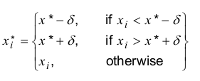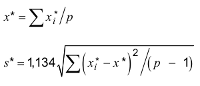# Welcome to the South African Wine Laboratories Association

## ISO 13528 Statistical Methods for Inter laboratory Proficiency Testing

### Robust Analysis Algorithm A is applied

This algorithm yields robust values of the average and standard deviation of the data to which it is applied. It was reproduced from ISO 5725-5.

Robustness is a property of the estimation algorithm, not of the estimates it produces, so it is not strictly correct to call the averages and standard deviations calculated by such an algorithm robust. however, to avoid the use of excessively cumbersome terminology, the terms "robust standard deviation" should be understood in this International Standard to mean estimates of the population mean or of the population standard deviation calculated using a robust algorithm.

Denote the p items of data, sorted into increasing order, by:

x1, x2, ..., xp

Denote the robust average and robust standard deviation of these data by x* and s.

Calculate initial values for x* and s* as:

x* = median of xi   (i = 1, 2, ..., p)

s* = 1,483 median of |xi - x *|   (i = 1, 2, ..., p)

Update the values of x* and s* as follows. Calculate:

δ = 1,5s*

For each xi (i = 1, 2, ..., p), calculate:Calculate the new values of x* and s* from:where the summation is over i.

The robust estimates x* and s* may be derived by an iterative calculation, i.e. by updating the values of x* and s* several times using the modified data, until the process converges. Convergence may be assumed when there is no change from one iteration to the next in the third significant figure of the robust standard deviation and of the equivalent figure in the robust average.
Events